telescopeѲptics.net          ▪▪▪▪                                             CONTENTS

10.2.1.1. Hamiltonian telescope       10.2.1.3. Honders camera

# 10.2.1.2. Schupmann medial telescope

Toward the end of 19th century, catadioptric dialytes were to enlist a new design, whose originality and high level of correction will secure for it a special place and respect up to this day. After years of work on finding solution to the main problem of dialyte objectives - excessive lateral color - German optician Ludwig Schupmann had it finalized in the form of his "medial" (cross between refractor and reflector) telescope. All it took were two seemingly simple steps. The first was to let the front lens focus, and place the second, catadioptric element (CE, which is a subaperture Mangin mirror) in its diverging light. The second step - a stroke of genius - to place an accessory lens at the front lens' focus.

This little lens did the impossible: by forming the image of the front lens in the continuum of optical train, it has brought their chief rays where they needed to be in order to cancel lateral chromatism - to the center of this image. Simply by placing the CE at the location of this image, its exit pupil is back on its surface, and lateral color disappears. At the same time, it also brings back together wavelengths widely scattered by front lens' primary spectrum (its longitudinal chromatism). However, that itself doesn't correct primary spectrum of the front lens, since rays for different wavelengths are still at an angle, and need to exit the last element not only close together, but also nearly parallel in order for the longitudinal chromatism to be cancelled. Since the CE has even more important task of correcting spherical aberration of the front lens, its power needed for one of the two tasks will not coincide with the power needed for the other one, except for one particular CE separation, if both elements (the front lens and CE) are made of the the same glass type. This, single-glass Schupmann, will be analyzed hereafter.

For CE separations with which only one of the two aberrations can be corrected, it is longitudinal chromatism, while spherical aberration in such case has to be removed by aspherizing one of lens surfaces.

Obviously, since the CE forms the final image image at the focal plane of the front lens, in order to make the final image accessible the CE has to bi tilted. Aberrations induced by tilting CE are minimized by a compensatory tilt of the front lens.

The conventional starting configuration for the Schupmann medial telescope is with the CE focusing right back at the front lens' focus. As mentioned, for one particular CE separation both spherical aberration and longitudinal chromatism can be simultaneously corrected. In practice, good correction can be achieved in a short range of separations around this optimum separations, by way of relatively small adjustments in system parameters, that may include small deviations of the final focus location from its "default" location at a distance from the CE equal to the CE-to-front-lens-focus distance.

How does it actually work? After all mystique surrounding it, it may sound all too simple. Neither the small focal zone lens (or mirror, as it doesn't make difference what type of optical element re-images the front lens) nor the reflecting surface, for which its object (image created by the preceding surface) and image nearly coincide at its center of curvature, induce any significant aberrations, on- or off-axis, except for astigmatism induced by the latter. The aberration calculation practically reduces to the front lens (for object at infinity) and the refractive surface of CE (first refraction for object at the front lens' focus and image at the reflective surface's center of curvature, reversed for the second reflection).

In the described setup, the system is to a large degree self-compensating. Since the front lens induces significant spherical aberration, it needs to be offset by spherical aberration of opposite sign generated by the two refractions at the CE. For corrected coma the front lens needs to be coma-free (there is no appreciable contribution from the CE). Astigmatism before element tilting remains low enough not to command special attention.  Lateral color is corrected by the small pupil-imaging mirror, while longitudinal chromatism, as already mentioned, can be corrected for any CE separation, but there will be only one particular separation where CE will also be correcting for spherical aberration. The system focal length nearly equals that of the front lens.

The simple recipe for the Schupmann medial telescope is, therefore, nearly coma-free front lens of the focal length desired for the system, small concave mirror placed at the lens' focus, to re-image the front lens at a distance allowing for corrected both, spherical aberration and longitudinal chromatism, and catadioptric element (CE) placed at the location of re-imaged pupil, with the focal length nearly 1/2 of the mirror-to-CE separation, so that it focuses diverging light right back to its focus of origin.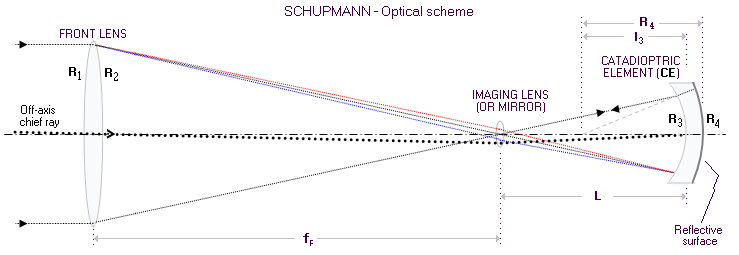FIGURE 165
: Optical scheme of Schupmann's medial telescope with main parameters. Power of the imaging lens/mirror is such that it forms image of the front lens (i.e. entrance pupil) at the front surface of the CE, by which it at this surface: (1) brings together rays of different wavelengths, and (2) brings together chief rays. of all wavelengths. If the reflective CE radius is such that its center coincides with the image of the imaging lens/mirror formed by the front CE surface, the rays of all wavelengths - which refract differently entering CE - are reflected back to the same point of entry at the front CE surface. Thus they follow the same path in reverse, focusing onto the imaging lens/mirror. For corrected longitudinal chromatism, the two refraction at the front CE surface need to compensate for the discrepancy in the incident angle at this surface, so that all wavelength are exiting CE not only at nearly the same point, but also nearly parallel. This will bi accomplished with a single specific combination of R
3 and R4., which exists for any separation L between CE and imaging lens/mirror However, spherical aberration can be simultaneously corrected only for a very narrow range of the separation, approximately equaling one half of the front lens' focal length. The farther from that location CE, the greater spherical aberration residual, which can only be corrected by aspherizing one of lens surfaces.
As for the lateral color correction, since the chief rays of different wavelengths are brought to the CE center, they are much less sensitive to the change in the radii values, and remain close (i.e. well corrected) after reflected back to the final focus.

With respect to the front lens, thin lens imaging object at infinity generates zero coma if its shape factor, defined as q=(R2+R1)/(R2-R1), where R1, R2 are the front and rear surface radius of curvature, respectively, relates to the glass index of refraction n as q=(2n+1)(n-1)/(n+1). Substituting for the front lens' index of refraction n gives its needed shape factor q1, in general a biconvex lens with the front surface radius of curvature significantly stronger than the rear radius. The value of q1 determines the R2/R1 ratio for the front lens as R2=(1+q1)R1/(q1-1), which after substitution in Eq. 1.2 gives

R1=2(n-1)ƒF/(1+q1)            (m)

ƒF being the front lens' focal length. Spherical aberration of this lens is given by Eq. 49.1, which further simplifies with n and q1 known. Taking n=1.52 for BK7 crown, and the corresponding shape factor for the coma-free front lens q1=0.83, gives as the corresponding aberration coefficient sF=1/3.9ƒF3. Substituting from the above relation ƒF=(1+q1)R1/2(n-1)=1.76R1 and

sF=1/21.3R13         (n)

The small concave mirror (as mentioned, it can also be a positive lens, but mirror produces more compact, practical system configuration) placed in the focal plane of the front lens only needs to fulfill one requirement, to re-image the front lens - at which all three, the aperture stop, entrance pupil of the system and exit pupil for the mirror coincide - at an arbitrary distance L. Since its object is the lens at a separation equal to F, needed mirror focal length is, from Eq. 1.4, given by

ƒM=LƒF/(L+ƒF)

doubled for the radius of curvature. Note that all three values here are numerically positive, due to Eq. 1.4 being not coherent with the sign convention; in the standard Cartesian coordinate system all three distances, being measured from right to left, are numerically negative. With this in mind, the needed radius of curvature of the concave mirror is RM=-2ƒM.

With lateral chromatism reduced to negligible at the exit pupil formed by the mirror, with near-zero coma and also negligible astigmatism induced by the front lens, the role of the optical element placed at the mirror's exit pupil is to correct lens' spherical aberration and primary spectrum, while inducing no other appreciable aberrations of its own. Turned out, a job tailored for a negative meniscus with its inner concave surface made reflective: the catadioptric element (CE). There is no strict requirement that the CE reflects light exactly back to the front lens' focus, but since nothing is gained if it doesn't, and convenience of the symmetry is lost, the concept of the two foci nearly coinciding is usually the most practical, at least as a starting point. For the two foci to nearly coincide, the rear (reflecting) surface needs to reflect light right back where it apparently came from, and that is toward virtual image produced by the refracting surface in front of it. In other words, center of curvature of the reflecting surface needs to coincide with the virtual image. This also cancels spherical aberration and coma at the reflecting surface, leaving in only its low astigmatism.

Thus, we have a fourth surface - the front, refracting surface of the CE - placed at an arbitrary distance L from the mirror in the focus zone of the front lens, that needs to offset spherical aberration generated by the front lens, while forming a virtual image that coincides with the center of curvature of the reflecting lens surface following it. For the first task, its aberration coefficient for lower (4th) order spherical for the front CE surface,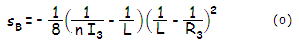needs to have such value that

2sBdC4=-sFdF4       (p)

where n is the refractive index of the rear element (assumed identical to the refractive index of the front lens), I3 the separation from the front CE surface to the image it forms (equal to the radius of curvature of the rear, reflecting surface R4 plus rear element's thickness), R3 is the third surface radius of curvature, dF, dC are the effective aperture radii for the front lens and catadioptric element, respectively, and sF the 4th order aberration coefficient for spherical aberration at the front lens (Eq. 50).

The sB coefficient is doubled because the aberration contribution from the second refraction at this surface is nearly identical when the light is reflected back at the same angle, thus doubling in the total of aberration. Expressing marginal ray height at the CE in units of the front lens aperture radius as dC, the two coefficients need to be related as 2sBdC4=-sF. Since the marginal ray height for green light at the CE's front surface is not appreciably affected by a reflection from the mirror in the focus zone of the front lens, it can be expressed as dC=L/ƒF, dC (as absolute value) is numerically identical to the relative CE separation from the front lens' focus in units of the lens' focal length.

Note that the mirror-to-CE separation L in the configuration with a small imaging mirror is, according to the sign convention, numerically negative, but as the surface-to-object separation for the front CE surface, used in Eq. (o), it is reversed, i.e. positive. Also, both CE radii and the distance from R3 surface to the image it forms, I3, are all numerically positive.

Unfortunately, there is no simple way to express the needed 3rd surface radius R3 in terms of other parameters. Since both, 3rd surface radius and its image separation are needed to calculate the aberration coefficient, process of iteration would require to calculate, from Eq. 1, the appropriate value

I3=nLR3/[(n-1)L+R3]             (q)

for every value of R3, until the values for which the two sides of Eq. (p) are nearly identical in their absolute value are found - if there is such. The sum residual of the two coefficients, Δs=2sBdC4+sFdF4, determines the system P-V wavefront error of spherical aberration at the best focus as W=Δs/4.

If aspherizing is an option, R3 value only needs to be determined solely to fulfill the requirement for corrected longitudinal chromatism. In an all-spherical system, the approximate R3 value for corrected longitudinal chromatsm can be used to narrow down the range of R3 values for which both of these aberrations are corrected, i.e. to determine its approximate starting value.

For corrected longitudinal chromatism, from ray geometry on FIG. 165, neglecting the effect of the small concave mirror, two refractions at R3 will compensate for chromatism generated by the front lens when R3=2(n-1)L/(2n-1) and L=ƒF, i.e. for the CE separation equaling the front lens' focal length. Since the incidence angles of blue and red rays increase in inverse proportion to the reduction in L, the needed value for R3 decreases by that much faster than L itself, and the general approximation can be written as

R3 ~ 2(n-1)L/(2n-1)         (r)

where =L/ƒF is L in units of the front lens' focal length ƒF. Since the mirror does change the geometry somewhat, the actual correcting value will be somewhat smaller for L=ƒF, gradually changing to somewhat larger value toward L=0.25ƒF. For the range of practical CE sizes, ƒF/2LƒF/3, the approximation in Eq. (r) will give usable starting R3 value.

With L in units of the front lens' focal length ƒF, the front CE radius, also in units of ƒF, is

R3~2(n-1)2/(2n-1)           (s)

For calculation purposes, it is convenient to express all relevant parameters in units of ƒF: substituting for L and R3 in units of ƒF in Eq. (q) gives

I3~ n2/(n+-0.5)          (t)

ƒM=ƒF/(+1)=L/(+1)          (u)

sF~1/3.9           (v),      and

sB~(1/8) [n+(2-n2)-1][(n-1)-n+0.5]2 / (n-1)2n26           (w)

Obviously, in order to come to their actual values, the relative values of R3 and I3 need to be multiplied by ƒF. Less obvious, sF and sB relative values need to be divided by ƒF3.

Since a common quality crown is the most likely glass choice, these relations can be further simplified by assuming n=1.52. With it, R3~2/2, I3~1.522/(2+0.52) and sB~(1/8)(0.52-0.31)(0.52-1.02)2/0.6256. These parameters now can be easily plotted.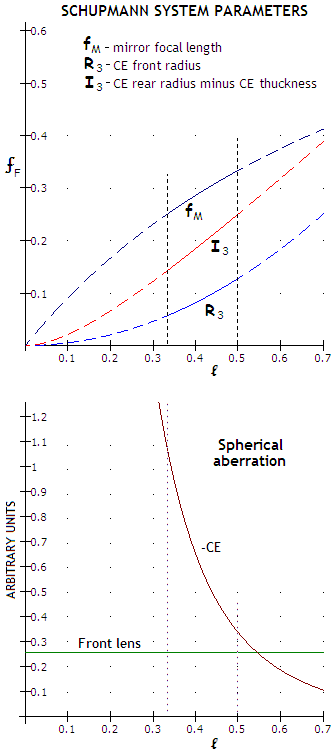On top, shown are approximate values indicating, directly or indirectly, surface radii fallowing the front lens, in units of its focal length: focal length of the small imaging mirror (ƒM), which is one half of its curvature radius, curvature radius of the front CE surface (R3), and image distance for this surface, which with the CE center thickness added to it equals the curvature radius of the rear, reflective CE surface (R4). All three are a function of the relative CE separation () from the front lens's focus in units of the front lens' focal length (ƒF); this is also, to a close approximation (slightly smaller), the relative size of CE in units of the clear front lens' aperture. These surface curvatures are needed to correct for longitudinal chromatism and lateral color in the conventional Schupmann scheme as described above. The only potentially significant remaining aberration before tilting is spherical.

Below are plotted spherical aberration contributions of the front lens and CE, also as a function of . Note that the sign of aberration generated by the CE is negative, opposite to that generated by the lens, but its sign is reversed for it makes it easier to compare the magnitude of two contributions. When they are numerically equal, the system spherical aberration is zero. As plots indicate, the corresponding value is about 0.55, i.e. with the CE placed at 0.55ƒF from the front lens' focus. The smaller CE, the greater contribution imbalance, due to the CE contribution increasing exponentially due to the significantly faster rate of decrease in R3 vs. rate of decrease in CE effective aperture. At the lower end of the range of practical CE sizes (~1/3), the CE contribution is roughly four times greater, and aspherizing would very likely be unavoidable. For instance, a 150mm ƒ/10 front lens generates 1.15 wave P-V of spherical aberration, which means that at ~1/3 the system error would be some 3.5 waves - likely too much to correct by readjustments (the actual aberration is obtained by multiplying "arbitrary unit" value with d4/4ƒF3, d being the aperture radius).

These approximations are best suited to the conventional arrangement on which they are based, i.e. with the small pupil imaging mirror in the focal zone of the front lens, and with the catadioptric element (CE) focusing back at the front lens' focus (before tilting the elements). As mentioned, functional system of this type can be arranged with relatively minor, but not negligible deviations from this original concept. The effect of moving the mirror out of the focal zone are:

(1) chromatic, due to the induced by it mirror power resulting in the change of relative ray heights at the CE for different wavelengths (moving the mirror closer shifts the blue closer, and the red farther away after reflection, relative to the green, and the other way around for moving the mirror farther away; it also diminishes and increases, respectively, lateral magnification in the blue/violet, and does opposite with the red, but this effect is comparatively insignificant), and

(2) small increase in spherical aberration generated by the CE when moving the mirror closer - due to the increased ray height at the CE - and decrease when moving it farther out.

Note that this assumes no change neither in the mirror-to-CE separation, nor CE surface curvatures. Given surface curvatures, the effect of moving the mirror can be neutralized by a change in the mirror-to-CE separation that will restore the original ray heights at the CE. Placing the small mirror slightly outside the front lens' focus has the benefit of avoiding re-imaging the mirror surface (i.e. possible surface contaminants and blemishes) into the final image. Such small shift from the original mirror position would require minor - but necessary - adjustment of the system parameters (keeping in mind that the front lens' focus is always at a little smaller separation from the rear lens surface than the nominal focal length).

The relations given above are all that is needed to put together an initial Schupmann system whose overall correction will be close enough for the final optimization with raytrace.

EXAMPLE: A 150mm ƒ/10 Schupmann medial telescope, with two BK7 glass elements (n1,2=1.52, V1,2=64.4 for the
e-line) and a concave mirror at the front lens' focus. The final system focal length equals that of the front lens, ƒ=ƒ
F, respectively. Starting with a coma-free front lens, the appropriate shape factor q1=(2n+1)(n-1)/(n+1)=0.83, determines the needed lens front radius R1=2(n-1)ƒF/(1+q1)=852mm, and its rear radius R2=(1+q1)R1/(q1-1)=-9172mm.

Needed radius of curvature of the small concave mirror placed at 1500mm from the front lens is RM=-2LƒF/(L-ƒF). Choosing for the lens' focus to the CE separation L=-ƒF/2=-750mm gives RM=-1000mm. For the first approximation of the front radius of the rear element, choosing the first round value above L/4 leads to R3~200mm. Radius needed for the minimized longitudinal chromatism is, from Eq. (r), R3~L/4~191mm. It suggests that R3~190mm is probably better a choice, but we'll proceed with R3=200mm, in order to find out how well this much of a discrepancy can be corrected by system re-adjustments.

The corresponding image formed by it is at a distance of I3=nLR3/[(n-1)L-R3]=-386mm (keep in mind that R3 in this configuration is numerically negative, and L is positive, so the quantities in the denominator add up). Choosing 10mm for the element's center thickness t3 (numerically negative from right to left), gives the radius of curvature of the reflecting surface R4=I3+t3=-396mm. The complete prescription in OSLO is: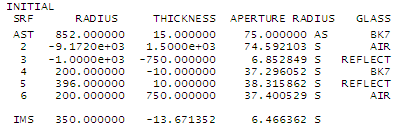This is all that is needed for the initial system layout. Plots below show that the correction level of monochromatic aberrations is excellent, and there is no noticeable lateral color. Longitudinal chromatism, on the other hand, while greatly reduced vs. that of the front lens alone (only about 20% greater than in a comparable doublet achromat), is still far from desired. As an illustration, it will be demonstrated how it can be minimized by the three methods mentioned above, without attempting to achieve best possible overall system correction (by the way, with R3=190mm and corresponding R4 value, longitudinal chromatism would have been practically cancelled, but the system would have 0.36 wave P-V wavefront error of lower-order spherical).

Probably the most convenient way of minimizing the longitudinal chromatism, if doable, is by adjusting the separation and radii of the CE, without resorting to a different glass type, or aspherizing. The limiting factor is spherical aberration induced by these changes. For this particular system, increasing the mirror-to-CE separation L from -750 to -777mm eliminated longitudinal chromatism, but also generated 1/5.6 wave P-V of spherical aberration. While it is still acceptable for most purposes - resulting in the Strehl just shy of 0.9 - it is beneficial to have it lowered to ~1/8 wave P-V. This is accomplished by a simultaneous increase of the R3 value by 3mm (to 203mm), reduction in R4 value by 1mm (to 395mm) and additional increase in the separation L to -790.5mm. The correction is overdone - as optical designers tend to do trying to reduce the ray spot to a point - since 1/34 wave P-V (1/114 wave RMS) of spherical aberration produced by it is well below the level when additional decrease in the size of aberration produces appreciable effect.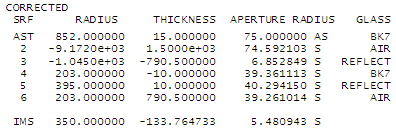As unavoidable effect of moving the CE away from the the image of entrance pupil formed by the concave mirror, lateral color is re-introduced; in order to have it cancelled, the pupil image - which is in effect the exit pupil for the CE - has to be brought back to it by extending small mirror's f.l. to 1045mm. Also, due to the relatively significant shift of the final focus toward the CE, the final focal length is reduced to 1250mm, for an ƒ/8.4 system.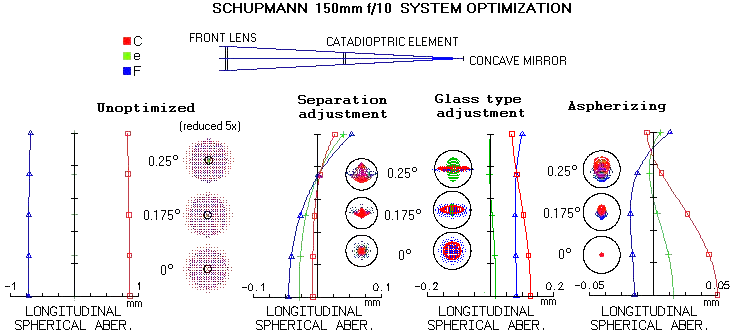Another option for balancing longitudinal chromatism and spherical aberration in the Schupmann is to use different glass types for the front lens and CE. In this case, switching from Schott BK7 to K5 crown (n2=1.525, V2=59.72) for the CE results in the longitudinal chromatism near cancelled, with only a small change in the CE separation L (6.3mm smaller) needed to bring together red and blue. At 0.16 and 0.2 wave P-V, respectively, the C and F lines are well within the "true apo" requirement (1/4 wave), however, combining two different glasses results in the introduction of secondary spectrum. The common C/F focus is shifted from the green focus, with the wavelengths beyond these two being exponentially more defocused, particularly toward violet. For instance, error in the violet g-line (436nm) is already over 0.8 wave (using another inexpensive crown, BALKN3, would reduce secondary spectrum by nearly one third, with only 1mm increase in CE separation needed vs. initial arrangement). While this low level of chromatism is still unnoticeable visually in most instances, a single-glass arrangement without residual secondary spectrum is generally the preferable choice.

Finally, longitudinal chromatism can be dealt with by slightly aspherizing the front CE surface R3. Adjusting R3 and R4 (to 190 and 383mm respectively) to correct for longitudinal chromatism, results in a nearly 1/2.5 wave P-V of spherical aberration induced to the system. It is reduced to a practical zero by putting -0.022 conic on R3 (surface 4 and 6 in the prescription). The only remaining aberrations are field curvature, insignificant in visual observing, and astigmatism, nearly entirely induced by the CA reflective surface. Obviously, aspherizing option is the easiest one for obtaining best correction in the design process; however, it's not likely to be from the standpoint of system fabrication.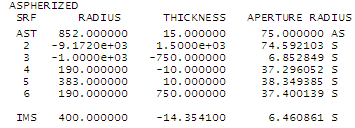The final step in designing the Schupmann is making the image accessible by tilting all three elements. Small concave mirror typically needs about 4 degree tilt, catadioptric element less than half as much (it is not affected by the former), and the front lens about as much as the CE. Tilting the mirror doesn't induce appreciable aberrations, but tilting the CE does. This is why the front lens needs to be tilted as well, in the plane perpendicular to CE's tilt, in order to offset astigmatism induced by tilting the CE. If done correctly, tilting will not appreciably affect the mid-field aberration level. It does, however, increase off-axis astigmatism, and produces asymmetric, tilted image field typical for tilted systems. It also presents an additional difficulty in achieving and maintaining proper system alignment.

Tilting the elements also reveals the effect on the final performance of above deviations from the basic concept of coincident foci in order to achieve simultaneous correction of spherical aberration and longitudinal chromatism with CE separations different than the optimal.

Tilting the elements as needed for making the final image accessible in the axial system corrected (primarily) by the CE separation adjustment, shows that there is a price to pay for the final focus being significantly closer to the CE than the front lens' focus. If we take the system corrected by axially adjusted element separation and tilt the elements as needed to make the final image accessible, the consequence of the significantly shorter focus of the CE is center-field coma that cannot be removed (TOP). With the CE image and object coinciding, there is a zero coma in the image. The more displaced the image from the object - which is here the image formed by the front lens - the more of off-axis coma is reintroduced into the CE image field. Tilting the CE then transforms this coma into the typical misalignment all-field coma, affecting evenly the entire field. In this particular case, the center field coma is still within "diffraction limited" 0.0745 wave RMS, but clearly not an appealing design optimum. Also, the shorter final beam requires larger CE (and, consequently, front lens') tilt, resulting in the larger off axis astigmatism as well.

As the enlarged area around small concave mirror shows, when at the nominal focal length (1500mm) behind the front lens' rear surface, the mirror is slightly (nearly 10mm) behind the lens' focus, hence the reflected cone is actually slightly slower than ƒ/10. However, it is the CE radii required to have both, spherical aberration and longitudinal chromatism corrected, that determine the final, faster focal ratio.Better alternative to correcting the initial Schupmann system by increasing the CE-to-lens'-focus separation alone is to combine the separation increase with reduction in the lens-to-mirror separation (MIDDLE). With the mirror shifted in front of the focus, the reflected beam is now slightly wider (faster), and the CE aperture needed for corrected spherical aberration is reached at a smaller focus-to-CE separation. Due to the changed geometry of rays, the CE radii needed for the complete correction now reduce the final focal ratio significantly less, to ƒ/9.4. More importantly, the CE separation from the two foci is nearly identical, resulting in zero coma. Off axis astigmatism is smaller due to the smaller tilt required for a similar final focus clearance. Lateral color error is  minimized, as before, by a small adjustment in the mirror radius and CE (surface 4) decenter.

Finally, a system built starting with somewhat larger CE, as indicated by calculation to be needed for near zero system spherical aberration (BOTTOM). The lens-to-mirror separation is the same as in the top example, CE is nearly two inches farther from the front lens' focus, and the final focus is nearly in the same plane as the small concave mirror, but the design performance is nearly identical to that of the middle arrangement. The final image is tilted some 4 degrees clockwise with respect to the central cone axis. The -2.5° tilt in the prescription is compensating for the tilt of the mirror and CE, which add up to 4.9° image tilt relative to the central-cone axis, but it is with respect to the sagittal astigmatic image surface; best image surface lays midway between sagittal and tangential surface, and with the latter being closer to the CE, the actual tilt of the best surface is always smaller than the sum of the small mirror and CE tilt angles (tilt shown in the prescription is in the tangential plane, the one determined by the axis and chief ray; due to the tilt of the front lens, the final image is also tilted in the sagittal plane, which will be shown in the following more detailed analysis of this last system).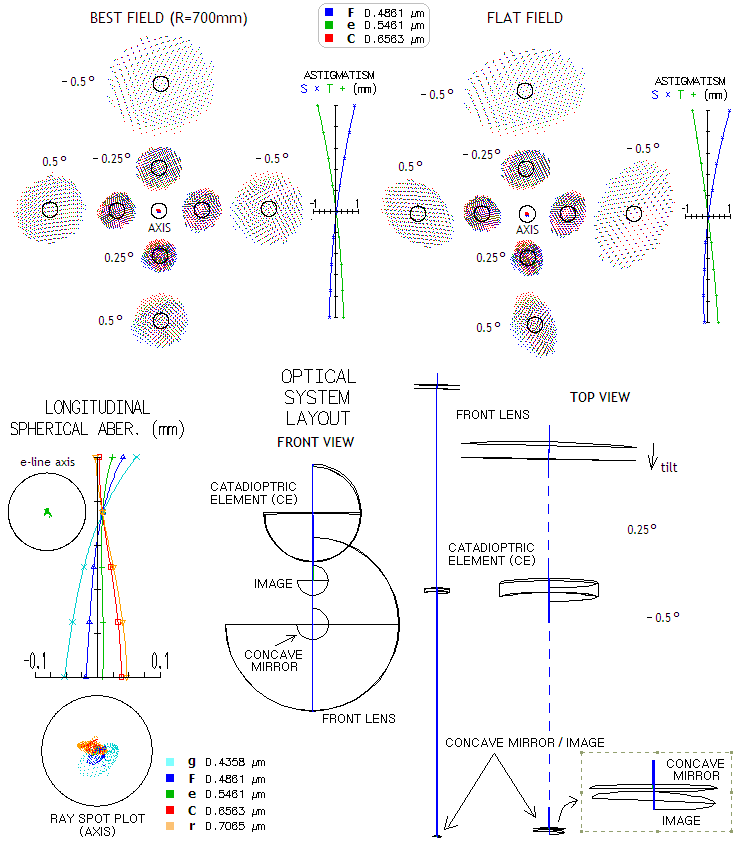As the plots show, best image field is not flat, with the best field being concave toward eyepiece, with 700mm radius of curvature. However, due to the strong off axis astigmatism, ray spots are not significantly larger over flat field, and the moderate best image curvature should allow efficient eye accommodation over the best image field (in other words, eye will see the best field). Due to the final field being effectively a sum of the two imperfectly matched fields, that of the front lens, and that of the CE, it shows asymmetry in its field astigmatism, with the upper field having significantly stronger astigmatism than the lower field, with the side fields astigmatism being approximately in between (note that due to the system forming the final image from a diverging cone, the sign in the image space is, like in the Gregorian system, reversed). Also, due to the astigmatism on two opposing field sides having sign reversal, half of the field will have astigmatism of the same sign as that of the eyepiece, hence adding to it, and the other half will have it of opposite sign, making it smaller.
The flat field ray plots show additional field asymmetry arising from the best image surface dual tilt, due to the two tilted elements being tilted in the planes perpendicular to each other (shown enlarged bottom right). Longitudinal aberration plot and ray spot plots bottom left show that the design achieves exceptionally high level of axial correction, both mono- and polychromatic.

The last important aspect to address is the system sensitivity to misalignment, i.e. tolerance level for misalignment errors. The simplest way to asses it is to determine how much of tilt error at each element results in the maximum acceptable axial aberration, here taken to be the conventional "diffraction limited" level, i.e. 0.074 wave RMS wavefront error (0.80 Strehl) in the central wavelength. Other two elements are assumed to bi in proper alignment (in the actual system, of course, they may not be, and it can either decrease or increase the final error).

 ELEMENT TILT ERROR INDUCING 0.075 WAVE RMS (degrees) FRONT LENS 0.13 SMALL CONCAVE MIRROR 0.085 (0.016) CATADIOPTRIC ELEMENT 0.13

For comparison, 0.13° misalignment sensitivity is comparable to that of a ƒ/7.8 paraboloid (mirror tilt sensitivity, several times greater than the diagonal tilt sensitivity). Tilt sensitivity of the small imaging mirror is somewhat higher at 0.085°, but even that would be far from acceptable due to the magnitude of accompanying lateral color error (due to tilt of this mirror effectively causing decenter of the CE vs. diverging cone). The C/F split in the field center is 0.035mm, or 2.6 times Airy disc diameters in the e-line. Since the maximum for this aberration is approximately one half of the Airy disc radius (for 0.80 photopic Strehl, FIG. 72), the lateral color tolerance for this mirror is five times smaller, or 0.016°. From Eq. 73.1 the corresponding paraboloid's F-number for given tilt t in degrees, RMS wavefront error ω and aperture diameter D(mm), is F=(tD/4.3ω)0.5, which for t=0.016, D-150 and ω=0.0745 gives F=2.75. Hence the only tight tilt error tolerance for the Schupmann system is for this small mirror, for this particular system comparable to the tilt tolerance for an ƒ/2.75 paraboloid. In this case it is causing all-field lateral color error lowering the photopic Strehl to ~0.80 in the field center.